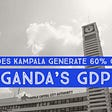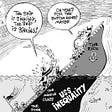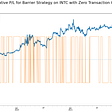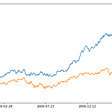# Think Like a Market Maker — Understanding Implied Volatility

Any option trader who focuses on volatility trading will tell you that the price of volatility is the single-most-important factor in trading volatility. That might sound intuitive to us, but it wasn't too long ago when implied volatility was merely a plug-number when we wanted to price an option (or just another one of the 6-factor model we all know as the Black-Scholes option pricing model).

Ever since I joined the market (about 15-years ago), I have been fascinated by the idea of implied volatility. How can we accurately forecast future volatility? Should we use historical price dynamics to predict future dynamics ( *cough..* GARCH/ARMA models)? How do we account for future events when we use historical volatility?

All of these questions pushed me to try and understand the nitty-gritty of how market-makers price volatility. It took me many years of trials and errors in attempts to reverse engineer prices and endless discussions with sell-side traders (and quants) to learn how market-makers view implied volatility.

When we price implied volatility, we can think of it as building blocks (or weights) that we add to our base volatility, which is our best estimation/guess of (future) realized volatility. Let's first define the different factors that affect the pricing of implied volatility:

1. Liquidity
2. The weekend effect
3. Seasonality
4. Economic/Earning calendar
5. Hedging flows

Now that we understand which factors go into the volatility pricing, let's dive into each aspect

# Liquidity

Most of us take liquidity for granted. We pay little to no attention to this factor, mainly because the trade sizes that we execute normally are a fraction of the available liquidity of the market we trade at any given point in time. Let’s say that we want to trade 20 E-mini contracts/ 10mio EURUSD / 100 TY Futures. These trades will hardly move the market because of the depth of these markets. For market markers and large investors (Hedge funds, Institutional investors, HFT firms, etc..), liquidity means the ability to carry on their day-to-day trading activity with minimal transaction cost and market impact. When a market maker runs an options book, liquidity plays a major role in the volatility price setting. As the dealer needs to continuously hedge the delta risk (i.e., needs spot liquidity) and occasionally unwind options (either in the market or at the interbank brokers), these different liquidity needs will be factored in when the dealer quotes implied volatility. The more liquid the asset, the tighter the bid-ask volatility is. Furthermore, the more liquid the asset is, the lower the weight that the dealer will add to the base volatility.

To emphasize the liquidity-volatility relation, let's look at the bid-ask spread in GBPUSD vol scattered against the implied volatility itself. If we assume that the change in the bid-ask spread represents the level of liquidity (or liquidity conditions), we can definitely infer that it's correlated to the change in implied volatility.

# The Weekend Effect (aka. weekend premium)

Weekends (and non-trading days) have a meaningful effect on implied volatility pricing. As options are priced using calendar days (i.e., 365 days/year), we need to account for non-trading days (as there are roughly 250 trading days/year on average).

The mismatch between calendar days/trading days has two notable effects:

1. Implied volatility discount over weekends/holidays
2. Price discontinuity (weekend gap)

## Implied volatility discount

When we price short-dated options (let's take, for example, a Monday expiry on Friday afternoon), we need to account for the fact that despite the option being a T-days option, we have (T-t)-days of 0 volatility (where "t" is the number of net trading days), so effectively our vol should be

Let's look at a numerical example: It's a Friday, and we want to sell an option that expires on Monday (to collect decay over the weekend). Yesterday (Thursday), the implied overnight volatility was 16. We ask our broker for a quote on the 3-day option, and we get quoted 9.5vol. At the start, it looks as if our broker is trying to rip us off!. Yesterday, the o/n vol was 16, and today it's 9.5??? But if we understand the weekend effect, we can actually see that the "fair" quote would have been 9.25 (sqrt(256/3)), but the dealer added some risk premium (we will touch on that later).

Now, we don't want to sell such a low vol. We want to have a "chunkier" volatility premium, so we decided to sell a 1-week option (expiring next Friday) and maybe close it on Monday after collecting the premium.

We, again, ask our broker for a quote, and we get quoted 14vol. This looks to us like a better deal, so we sell the option, hoping nothing will happen over the weekend so we could close it first thing Monday morning. Following Monday, we asked our broker for a quote on the reminder 4-day option, but now we get quoted 16.8vol!. After expressing our dissatisfaction that nothing happened over the weekend, but the price got bumped, our broker calmly says:

"When you sold the 1-week option at 14, the first three days were priced at 9.25, so your forward vol implies 16.6 (so I just marked it 0.2vol higher)."

This example is crucial in understanding the concept of "forward-volatility" (or volatility term-structure). Given that we know the term structure's shape (and levels), we can calculate at t=0 the forward implied volatility for any point along with the term structure. Generally speaking, we can use the following formula to calculate the forward implied volatility

So we see that dealers discount non-trading days when pricing options (and volatility), but non-trading days impose another issue, which is price discontinuity and gap risk (at the open the following trading days). As we know, a lot can happen in 48-hours, so obviously, one cannot ignore that when pricing volatility and should weigh some likelihood of a gap at the open. Historically speaking, gap risk is priced at a premium, very much like volatility risk.

Historically speaking, Selling the gap risk tends to be a systematically profitable strategy, as there are rarely gaps over weekends (or at least on a close-close basis, there have been very few tail events over the last ten years).

If we examine our usual G10 risk proxies (like USDJPY or AUDJPY), we can definitely see that the weekend "gap-risk" is massively overpriced.

In fact, if we ran a volswap-like strategy where our realized vol is calculated off the NY close on Friday and the Wellington open on Monday, we would have accumulated a loss of ~2500 vol pts since 2016. Even with high-frequency volatility estimators, we would have still lost money being long overnight vol over the weekend in AUDJPY (and it doesn't really get much better if we examine other G10 currency pairs). That said, there seems to be some profitability in trading various FX currency pairs on a high-frequency basis, but that's not related to the "gap-risk" on the Monday open.

As we can see, the weekend effect (and the "gap-risk" following the weekend) are structural premiums that the options market uses to account for non-trading days (and discontinuity in price action, where traders cannot react in realtime to new information)

# Seasonality

Volatility, like most other statistical measures, tends to exhibit seasonality patterns. Part of the seasonality can be attributed to market microstructure and hedging flows related to known cycles in financial markets (Just think about the SPX/VIX expiration cycles and their effect on implied volatility and realized SPX volatility). Other factors that can affect seasonality in implied volatility are forecasted seasoned with heightened/depressed volatility (earning season and summertime come to mind when thinking about cycles of realized volatility). If we take a look at USDZAR, for example, we can definitely spot a strong negative seasonality for the implied 1-month vol over the month of July, where implied vol tends to sell-off into the summertime.

Obviously, we should ask ourselves whether this negative seasonality in implied is justifiable, or, in other words, is selling 1-month vol into the summertime in USDZAR a profitable trade. Let's examine this strategy using a theoretical short vol-swap position of the average 1-month vol in July compared to the average realized vol in August. We can see that realized vol in August tends to outperform the implied 1-month in July (dated back to 2008).

On the other hand, if we examine a close relative or USDZAR — the USDMXN, we can see that seasonality in implied volatility translates into lower realized volatility the following month, which makes the "selling-vol-into-the-summertime" strategy a profitable strategy.

Now, obviously, the strategies here are a bit biased, as we assumed that the vol-swap we traded represents the AVERAGE 1-month vol in July and was closed against the AVERAGE realized 1-month vol in August. Still, we can definitely see that the seasonality weighting in USDZAR is far from being reflected in the realized volatility the following month.

# Economic/Earning Calendar

The economic/earning calendar is probably the key factor in pricing implied volatility. Assuming that volatility (and noise in price action) is a function of new information received by the market players (and the reaction function to the degree of "data surprise"), one can understand why it’s crucial for traders to be familiar with the economic data/earnings calendar. Before we dive into the nitty-gritty of eco data analysis, we should acknowledge that not all data releases are created equal, and some data releases have a more meaningful impact on the market than others. Also, the reaction function to data release is highly dependent on the "market narrative," which makes historical data analysis not as straightforward as one might expect.

Calculating the "event weights" can be done in many ways, and each trader has their own way to analyze the weights, but the general idea is pretty simple:

1. Rank the different economic data releases by importance. For me, monetary policy-related events like CPI, labor market, and CB meetings are the most important, followed by growth data (like GDP and Retail sales) and economic activity (PMIs).
2. Calculate the historical weights for "event days" volatility. That can be done using the ratio between the 1-day volatility (or the average between the 1-day OHLC volatility and the 1-day volatility the next day, as the market digests the data) and the realized vol of the reference period prior to the data release (can be either 1-week,2-week realized volatility ex. the event). One can use conditional volatility to better estimate the probability distribution (given an upward/downward surprise).
3. apply the weighted-average "event-weight" for each event to the future economic calendar

Let's run this analysis to estimate the "event-weight" of US CPI for USDJPY volatility. First, let's plot the distribution of "event-weights" as produced using the above methodology.

As we can see, ON AVERAGE, events had a vol seasonality of about 2x the realized vol of the prior 1-week, which means that, based on historical data, CPI days tend to be more volatile than "non-CPI days."

Now, we want to assess the conditional volatility given an upward/downward economic-data surprise (as we have no clue prior to the release whether data will beat or miss the market expectations…). As we can see on the right chart, we should expect above-average realized volatility (compared to the already upward biased realized volatility on CPI days) both for a downward and upward surprise (although a downward data surprise tends to produce more volatility).

If we compare that to the implied overnight vol for CPI days, we can clearly see that the market markers assign about 2.2x volatility over the CPI event, which aligns with historical CPI events.

# Hedging flows

The last piece of the "implied volatility" puzzle is probably the hardest to gauge yet touches on the very basics of derivatives trading(and options in specific), and that's the usage as hedging instruments. As options (among other derivatives) are used as insurance for large portfolios of different underlying (from stocks and bonds to commodities and currencies), their value reflects some degree of "risk premium". Hedging flows control all three aspects of the volatility surface:

1. The level of the front end ATM volatility level (relative to realized volatility)
2. The shape of the volatility term-structure
3. The shape of the volatility smile/skew (and implied/risk-neutral probability density)

## ATM volatility level

The ATM volatility level is mostly used to gauge the "volatility risk premium", or, in other words, the excessive premium option sellers demand the options. The question we need to ask ourselves is — "exessive premium relative to what?". Most practitioners will compare the implied volatility for period T to the same length of the historical volatility window (for example, the implied 1-month vol to the realized vol of the last 1-month). This, inherently means that we assume the future underlying dynamic will be similar to the historical/realized price dynamic.

Historically speaking, implied volatility tends to trade at a premium to the future realized vol (which is why "short vol/gamma" is, systematically, a profitable strategy). To examine this hypothesis, we will run the following backtested strategy:

Short 1-month VolSwap on different asset classes proxies (and a multi-asset portfolio), rolled monthly. As we can see below, even with the negative performance Feb-March 2020, this strategy was profitable over the last 10-years

If we dive deeper into the data, we can definitely see that March 2020 marked a change in the correlation regime between different asset classes

Obviously, many multi-asset vol funds, which were a significant driving force in the volatility space, got liquidated and washed out, contributing to the decorrelation in implied volatilities across the different asset classes.

## The shape of the volatility term structure

After understanding the flows' impact on the front-end volatility risk premium, we should understand how the hedging flows affect the shape of the volatility term structure.

Generally speaking, the shape of the volatility term structure reflects the implied volatility mean-reversion tendency (i.e, the long-dated volatility less affected by short-term price dynamic) and is driven both by hedging flows by large institutional investors (that protect their "long carry" investments with option buying programs), and by flows from structure products (mostly yield-enhancers that are packaged as a note with embedded optionality).

## The shape of the volatility skew/smile

The implied volatility skew has been in the center of derivatives research since the late 70s/early 80s when practitioners questioned the validity of Black-Scholes' flat volatility across strikes/maturities assumption was introduced in their Nobel-Prize winning options' pricing formula.

Before we try to understand why volatility skew exists, let's first understand the idea behind the notion of volatility skew.

In the "naive" Black-Scholes volatility universe, we assume a bell shaped-like probability density. This means, basically, that our risk-neutral probability assumes a normal distribution of future outcomes. When we apply the skew (i.e., assuming a higher vol for one side over the other), our risk-neutral probability will become conditional on the level of spot/strike.

Does it mean that we really think that the spot has greater chances of going up/down? Not necessarily, but investors/dealers/market-makers will be willing to pay/demand an additional premium for the OTM options

So why does volatility skew exist?

We can give many reasons why the volatility level is not constant across different strikes. The volatility skew/smile shape is mostly driven by hedging flows and market perception regarding the covariance of spot-vol. In the equity space, for example, volatility of downside strikes in the indices (like SPX) priced at a premium relative to the equivalent call-moneyness (for example 5% OTM Put compared to 5% OTM Call). As large institutional investors (like pension/endowment funds ) are naturally biased toward long equities (as it carries, on a long horizon, good risk-adjusted returns), they are willing to pay an extra premium to protect their books against equities downturn or engage in "call overwriting" strategies, in which they hold the underlying asset, and sell calls to collect premiums. In both strategies, the premium for the call comes under pressure, while puts maintaining a premium over the ATM vol.

## A toy model to evaluate implied volatility.

So far, we've seen various factors that affect the pricing of implied volatility. As we would like to have the ability to assess whether the current pricing of implied volatility for a specific maturity is rich/cheap, we would like, ideally, to have a simple model that generates the "fair" implied volatility for a given maturity.

When we value the implied volatility, we need a few parameters:

1. The average "clean" realized vol of the recent Nth days (by clean, I mean realized vol of days that had no scheduled eco events) — We can use a combination of intraday (high-frequency) / Close-Close vol to get the average vol.
2. The scheduled data releases and their calculated weights — for example, If we have in our future term 2 CPI releases, and currently we value the weight as 2, we will assign the CPI a factor of 4 (N*W, where N is the number of events and W is the weight)
3. The seasonality factor for the expiration month of the year
4. The liquidity factor — -The more liquid our underlying, the lower the factor. IRL, the liquidity will also affect the bid-ask spread the market-maker will apply to the mid volatility
5. The number of calendar days (not business days) in our term

If we put it all together, we can generally arrive at the following formula

Note- we are dividing our weighted variance by the calendar days to account for non-trading days (i.e., "weekend effect").

Obviously, a few parameters here are widely unknown (the base realized vol, to which we apply the different weights and the different event weights). Still, many attempts to reverse engineer quoted prices yielded a very similar formula, where the length of the "base" volatility is roughly half the implied period (for example, using 2-weeks "clean" realized vol to arrive at the implied 1-month).

I hope my attempt to shed some light on how dealers/market-makers calculate/make volatility prices managed to give you some clue about the different factors that play a role in the pricing process.

As always, feel free to share your thoughts and feedback.

Harel.

--

--

--

## More from Harel Jacobson

Love podcasts or audiobooks? Learn on the go with our new app.

## LEAKS AND TAKEOVERS## Is Kampala Responsible For 60% Of Uganda’s GDP?## Perils of a Financialized Economy## Without Rules, Fiscal Policy Chaos Will Continue## Deal Breakers for Real Estate## Are Gold Miners Making Money at Newmont Mining Corp?## Optimizing a Trading Strategy without Backtesting the Alpha## The Gamma Exposure Trading Strategy.## Modern Trends in Financial Engineering## Buy on gap strategy and its performance over time